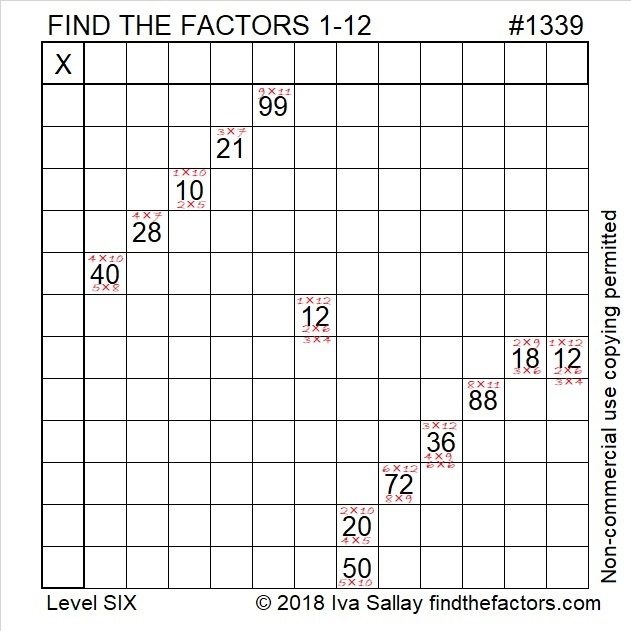# 1339 Written Eligible Factor Pairs Can Reveal the Needed Logic

The logic needed for this particular level 6 puzzle is VERY complicated so I wrote out the eligible factor pairs of all the clues for you.Or you can start from scratch:Print the puzzles or type the solution in this excel file: 12 factors 1333-1341

Here are a few facts about the puzzle number, 1339:

• 1339 is a composite number.
• Prime factorization: 1339 = 13 × 103
• 1339 has no exponents greater than 1 in its prime factorization, so √1339 cannot be simplified.
• The exponents in the prime factorization are 1, and 1. Adding one to each exponent and multiplying we get (1 + 1)(1 + 1) = 2 × 2 = 4. Therefore 1339 has exactly 4 factors.
• The factors of 1339 are outlined with their factor pairs in the graphic below.1339 is the hypotenuse of a Pythagorean triple:
515-1236-1339 which is (5-12-13) times 103

This site uses Akismet to reduce spam. Learn how your comment data is processed.